# Related Videos

## Simplify the expression $\frac{x^5-x^4+x^2-2}{x^2+1}$

Go!
1
2
3
4
5
6
7
8
9
0
a
b
c
d
f
g
m
n
u
v
w
x
y
z
.
(◻)
+
-
×
◻/◻
/
÷
2

e
π
ln
log
log
lim
d/dx
Dx
|◻|
=
>
<
>=
<=
sin
cos
tan
cot
sec
csc

asin
acos
atan
acot
asec
acsc

sinh
cosh
tanh
coth
sech
csch

asinh
acosh
atanh
acoth
asech
acsch

### Videos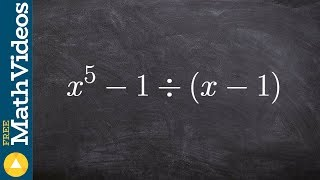### Algebra 2 - Learn how to divide using long division with multiple zeros, (x^5 - 1) / (x - 1)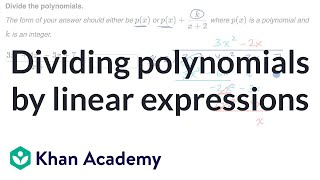### Dividing polynomials by linear expressions | Algebra 2 | Khan Academy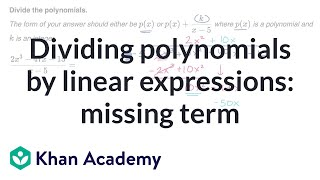### Dividing polynomials by linear expressions: missing term | Algebra 2 | Khan Academy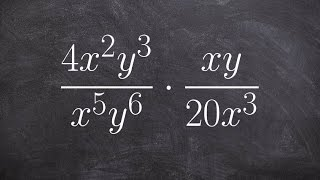### Algebra 2 - Simplifying the product of two rational expressions ((4x^2 y^3)/(x^5 y^6))*(xy/20x^3)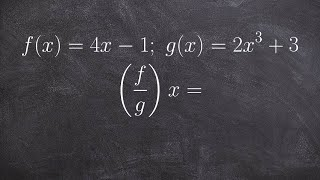### Algebra 2 - Find the domain from division of two functions, f(x)=4x -1; g(x)=2x^3 +3; (f/g)(x)=?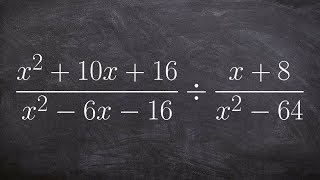### Algebra 2 - Divide two rational expressions by simplifying (x^2+10x+16/x^2‐6x‐16)/(x+8/x^2‐64)

$\frac{x^5-x^4+x^2-2}{x^2+1}$